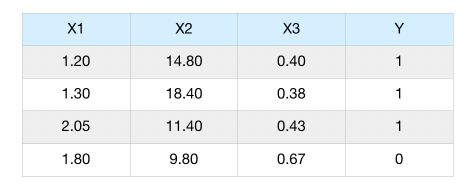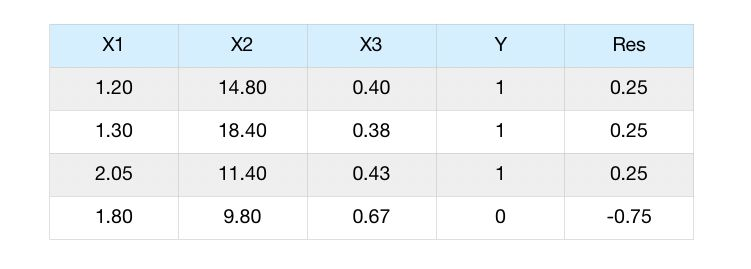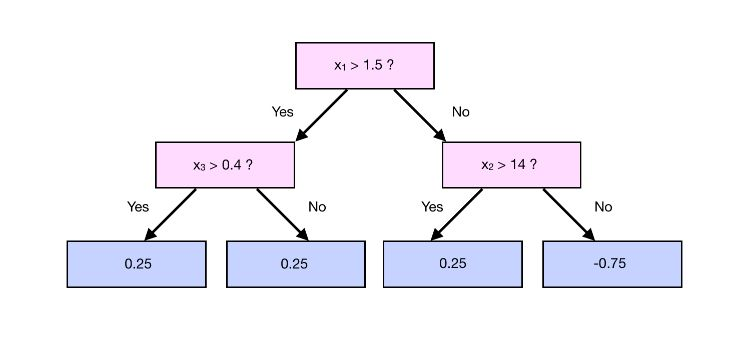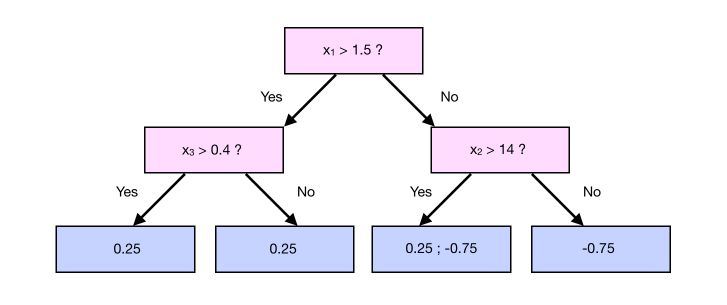Let’s consider a simple scenario in which we have several features, $$x_1, x_2, x_3, x_4$$ and try to predict $$y$$, a binary output.Step 1 : Make the first guess

The initial guess of the Gradient Boosting algorithm is to predict the log of the odds of the target $$y$$, the equivalent of the average for the logistic regression.

$odds = log( \frac {P(Y=1)} {P(Y=0)} ) = log( \frac {3} {1} ) = log(3)$

How is this ratio used to make a classification? We apply a softmax transformation!

$P(Y=1) = \frac {e^{odds}} {1 + e^{odds}} = \frac {3} {4} = 0.75$

If this probability is greater than 0.5, we classify as 1. Else, we classify as 0.

Step 2 : Compute the pseudo-residuals

For the variable $$x_1$$, we compute the difference between the observations and the prediction we made. This is called the pseudo-residuals.

We have now predicted a value for every sample, the same value for all of them. The next step is to compute the residuals :Step 3 : Predict the pseudo-residuals

As previously, we use the features 1 to 3 to predict the residuals. Suppose that we build the classification tree to predict the output value of the tree :In that case, we cannot use the output of a leaf (or the average output if we have more observations) as the predicted value, since we applied a transformation initiative.

We need to apply another transformation :

$\gamma_{i+1} = \frac { \sum_i Residuals_i } { \sum(\gamma_i \times (1-\gamma_i))}$

For example, take a case in which we have 1 more observation that falls into a leaf :In that case, the output value of the branch that contains 0.25 and -0.75 is :

$\frac {0.25 - 0.75} { 0.75 * (1-0.75) + 0.75*(1-0.75)} = -1.33$

Step 4 : Make a prediction and compute the residuals

We can now compute the new prediction :

$y_{pred} = odds + lr \times y_{res} = log(3) + 0.1 * -1.33 = 0.9656$

We can now convert the new log odds prediction into a probability using the softmax function :

$P(Y=1) = \frac {e^{0.9656}} {1 + e^{0.9656}} = 0.7242$

The probability diminishes compared to before since we had 1 well classified and 1 incorrectly classified sample in this leaf.

Step 5 : Make a second prediction

Now, we :

• build a second tree
• compute the prediction using this second tree
• compute the residuals according to the prediction
• build the third tree

As before, we compute the prediction using :

$y_{pred} = odds + lr \times y_{res} + lr \times y_{res_2} + lr \times y_{res_3} + lr \times y_{res_4} + ...$

And classifiy using :

$P(Y=1) = \frac {e^{y_{pred}}} {1 + e^{y_{pred}}}$

Conclusion : I hope this introduction to Gradient Boosting Classification was helpful. The topic can get much more complex over time, and the implementation is Scikit-learn is much more complex than this. In the next article, we’ll cover the topic of classification.

Categories:

Updated: